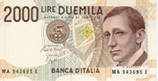Next: Exams Up: EE 163B: Communication Theory Previous: Homeworks

# HandoutsEE 163B

Matlab files and demos

1. DSB suppressed carrier demodulation using a Costas loop:

2. Intersymbol interference:
• Change the filter rolloff factor ("beta") in the R-C filter block and see the results.
• Observe the eye diagram for different values of beta. Is the eye "more open" when beta = 1? Can you see much less sensitivity to errors in the sampling times when beta is close to 1?
• Try increasing the noise level by changing sigma in the Rayl N block from 0.001 to 0.1. See how the scatter diagram smears, and also see the effect on the eye diagram.

EE 163A

Matlab files and demos

1. Quantization effects:
• Change the number of bits to R = 7, 6, 5, 4, 3, 2, 1 and listen to the output. You will notice that at a certain quantization level and signal-to-quantization-noise ratio the noise due to quantization becomes audible.
• You can also click below to hear the effects of quantization (to R bits or L = 2R + 1 levels) without running the program above: Original, 7 bits, 6 bits, 5 bits, 4 bits, 3 bits, 2 bits, 1 bit.

2. Matched filtering: